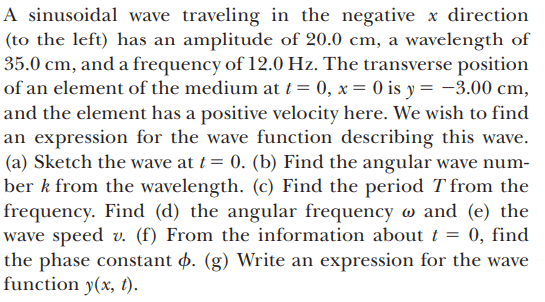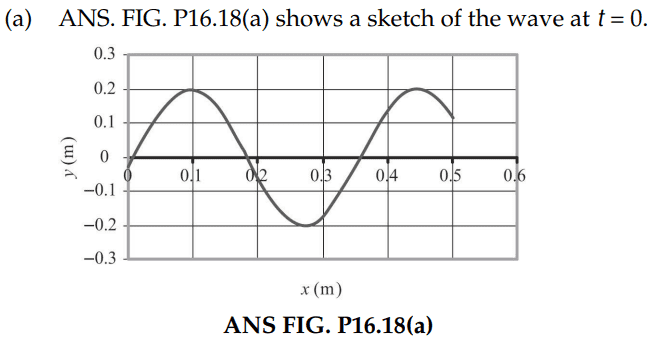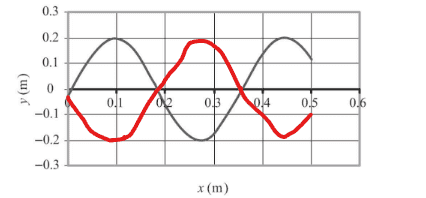# Graph for sinusoidal wave travelling to the left

Callumnc1
Homework Statement:
Relevant Equations:
For part(a) of this problem,The solution is,I don't understand why they assume on the graph where that the waveform is during it's phase. For example, could it not also be correctly drawn as shown in red:Could it not?

Many thanks!

#### Attachments

Gold Member
Not an expert on the subject, but you might want to reread the second sentence of the problem, again.

•Callumnc1
Gold Member
"... travelling in the negative x direction..." & "...the element has a positive velocity here."
What exactly do you think those mean in terms of y(0)?

•Callumnc1
Callumnc1
"... travelling in the negative x direction..." & "...the element has a positive velocity here."
What exactly do you think those mean in terms of y(0)?
Thank you for you reply @DaveE! I'm not sure what those means in terms of y(0)

Callumnc1
Not an expert on the subject, but you might want to reread the second sentence of the problem, again.
Thank you for you reply @hmmm27 !

Gold Member
Thank you for you reply @DaveE! I'm not sure what those means in terms of y(0)
OK let's consider a more concrete example. Imagine a surface wave in the middle of the ocean. You see a wave maximum moving from right to left. At a place you call "0" you drop a basketball. How does it move as the wave goes by.

•Callumnc1
Callumnc1
OK let's consider a more concrete example. Imagine a surface wave in the middle of the ocean. You see a wave maximum moving from right to left. At a place you call "0" you drop a basketball. How does it move as the wave goes by.

I think in an ideal case we assume that the ocean wave is traverse. Therefore, the basketball should not move since it should act as another element of the medium so will move vertically in simple harmonic motion with the other elements.

However, for a more realistic case, surface waves are made up of traverse and longitudinal waves, so the basketball will drift very slowly to the left in the direction of wave propagation along the ocean current.

Homework Helper
2022 Award
Consider only the transverse (vertical) motion of the basketball.

•Callumnc1
Gold Member
The point has a positive velocity. Will it’s value be larger, smaller or the same at the next instant in time?

•Callumnc1
Callumnc1
Thank you for your replies @hutchphd and @Frabjous !

So, if the basketball moves in transverse motion, then assuming the basketball is dropped at the wave maximum, then its transverse speed will be greater at the next instant of time.

Gold Member

I think in an ideal case we assume that the ocean wave is traverse. Therefore, the basketball should not move since it should act as another element of the medium so will move vertically in simple harmonic motion with the other elements.

However, for a more realistic case, surface waves are made up of traverse and longitudinal waves, so the basketball will drift very slowly to the left in the direction of wave propagation along the ocean current.
OK, so let's put some giant graph paper in the ocean. The vertical motion can be in the y direction, the direction of wave travel (perpendicular to the wave peaks) can be in the -x direction, because they said the wave moves that way. Now reconsider those two functions you drew. Which best matches what the basketball does?

•Callumnc1 and hutchphd
Homework Helper
Gold Member
2022 Award
To expand a bit on what others have said:

Remember the motion of an element at a particular x-position is purely transverse.

https://www.physicsforums.com/attachments/1675801695226-png.321914/

You are told that when at t=0. the element at x=0 has a positive (‘upwards’) velocity.

And you are told the waveform moves left.

Ask yourself (or sketch) where the red and black waveforms will be a short time later.

You should then see why the red waveform isn't correct but the black one is.

•Callumnc1 and hutchphd
Gold Member
Thank you for your replies @hutchphd and @Frabjous !

So, if the basketball moves in transverse motion, then assuming the basketball is dropped at the wave maximum, then its transverse speed will be greater at the next instant of time.
Is the wave higher or lower at the next instant in time?

•Callumnc1, DaveE and hutchphd
Callumnc1
•Steve4Physics, Frabjous and DaveE